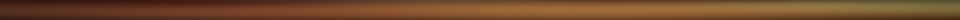# James Bremer

Department of Mathematics, University of TorontoMy work is principally concerned with the development of efficient algorithms for the numerical solution of elliptic boundary value problems and integral equations. Some of my recent projects include:

• A numerical algorithm for solving the two-dimensional Helmholtz equation in the special case of radially symmetric coefficients in time which grows linearly with frequency.
• A highly effective algorithm for numerical solution of the variable coefficient Helmholtz equation in one dimension which runs in independent of the frequency of oscillations of the solutions.
• High-order methods for the discretization of integral equations given on surfaces with complicated geometry, particularly those with edges and corners.
• O(1) algorithms for the numerical evaluation of a large class of special functions, which includes the associated Legendre functions, generalized Laguerre polynomials, Jacobi polynomials, etc.
• Fast algorithms for applying special function transforms, including the spherical harmonic transform and Jacobi transform.

Many of my software packages are available at my GitHub page.

#### Contact Information:

James Bremer
Department of Mathematics
University of Toronto
40 St. George St.
Toronto, Ontario M5S 2E4

email: bremer@math.toronto.edu
phone: (203) 843-4916